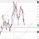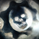2301 просмотров
2301
This creates a replica of relative distance from the moving averages, a good way to measure the strength, divergences etc.... short, mid and long term waves.

List of All my Indicators - https://www.tradingview.com/p/stocks/?so...
study(title = "Momentum Histogram", shorttitle="Mom Hist")

source=close
EMA_1=input(13, title="EMA 1")
EMA_2=input(21, title="EMA 2")
EMA_3=input(34, title="EMA 3")

hist1 = (ema(close,EMA_1)*close)-(ema(close,EMA_2)*close)
hist2 = (ema(close,EMA_2)*close)-(ema(close,EMA_3)*close)

Wave1=(hist1)
Wave2=(hist2)

plot(Wave2, style=histogram, color=red, linewidth=4)
plot(Wave1, style=histogram, color=#0066cc, linewidth=4)Hey, thanks for creating this indicator. Could you explain how you interpret the indicator, especially the light blue and red areas? Does the red mean the trend is slowing down, and blue confirms the trend?
ОтветитьVERSION - 2 Momentum Histogram Posted, follow Link -
ОтветитьI use those settings for Short term, Mid term and Long term. The red histrogram shows divergences. Only Blue, the trend is strong.
Ответить